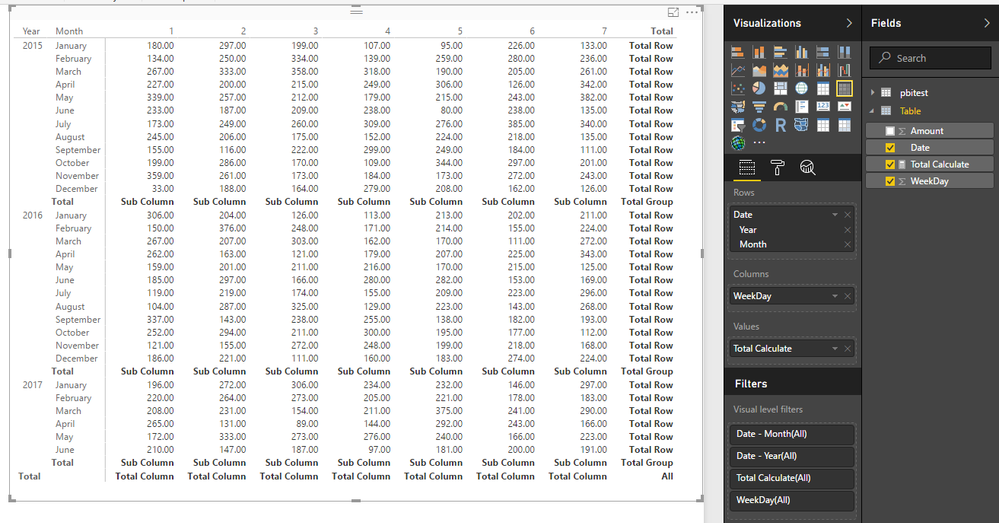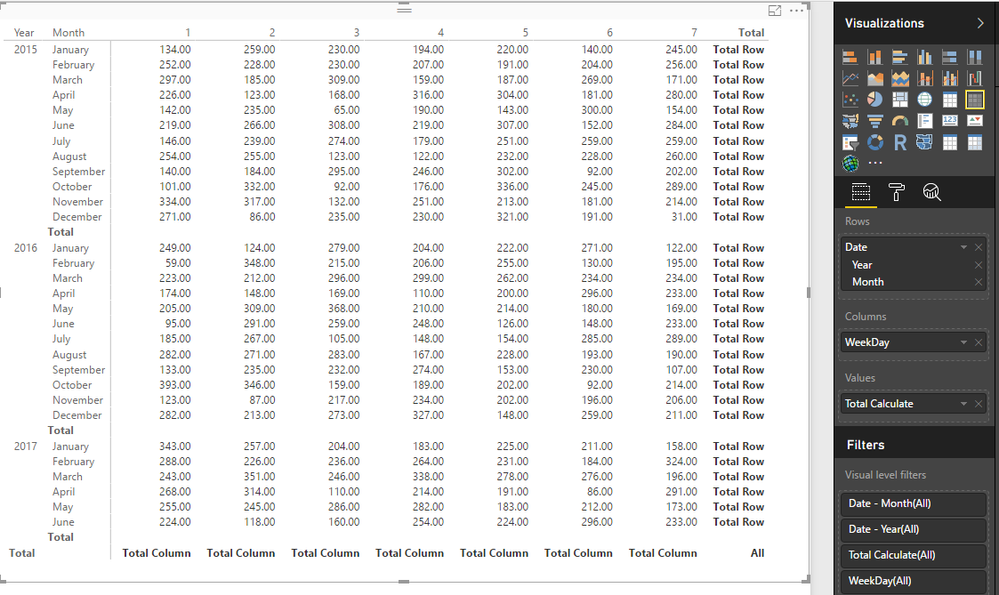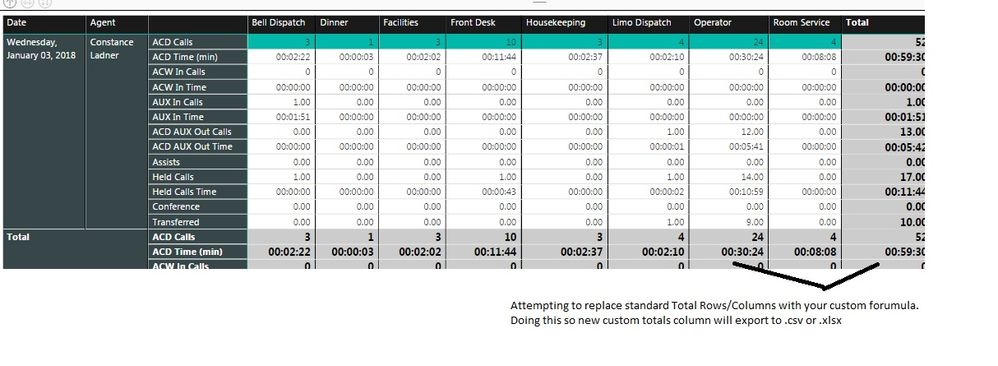cancel
Showing results for
Did you mean:Frequent Visitor

## Adding a custom total Row and Total Column for matrix

Hi all,

I am a new user of PowerBI and I have been trying to create a matrix with some data. I can get the value part of the matrix correctly (with 1s and 0s), but I am unable to add a total row and column at the end in the percentage format (this indicates the percentage of users who said a "1" out of the total answers we received.

I have attached a sample table created in excel (here 1- corresponding to "Yes" and 0 corresponding to "No", whereas a blank indicates no reply.

How should I go about it. Any help is appreciated.

Thanks,

S1 ACCEPTED SOLUTIONCommunity Support

Hi @sjain6,

Perhaps you can take a look at below formula if it suitable for your requirement:

Replace bold part with your calculate formula.

```Total Calculate =
IF(ISBLANK(MAX('Table'[Date]))=FALSE(),
IF(COUNTROWS('Table')=COUNTROWS(ALL('Table')),
"All",//all
IF(COUNT('Table'[Date])=COUNTX(FILTER(ALL('Table'),[Date].[Year]=MAX([Date].[Year])),[Date]),
"Total Group",//filter with gorup
IF(COUNT('Table'[Date])=COUNTX(FILTER(ALL('Table'),[WeekDay]=MAX([WeekDay])),[Date]),
"Total Column",//filter with column
IF(COUNT('Table'[Date])=COUNTX(FILTER(ALL('Table'),[Date].[Year]=MAX([Date].[Year])&&[WeekDay]=MAX([WeekDay])),[Date]),
"Sub Column",//filter with group + column
IF(COUNT('Table'[Date])=COUNTX(FILTER(ALL('Table'),[Date].[Year]=MAX([Date].[Year])&&[Date].[MonthNo]=MAX([Date].[MonthNo])),[Date]),
"Total Row",//filter with group + row
SUM('Table'[Amount])))))))```BTW, if you want hide some 'total level', you can use blank function to replace that part text.

```Total Calculate =
IF(ISBLANK(MAX('Table'[Date]))=FALSE(),
IF(COUNTROWS('Table')=COUNTROWS(ALL('Table')),
"All",//all
IF(COUNT('Table'[Date])=COUNTX(FILTER(ALL('Table'),[Date].[Year]=MAX([Date].[Year])),[Date]),
BLANK(),//"Total Group",//filter with gorup
IF(COUNT('Table'[Date])=COUNTX(FILTER(ALL('Table'),[WeekDay]=MAX([WeekDay])),[Date]),
"Total Column",//filter with column
IF(COUNT('Table'[Date])=COUNTX(FILTER(ALL('Table'),[Date].[Year]=MAX([Date].[Year])&&[WeekDay]=MAX([WeekDay])),[Date]),
BLANK(),//"Sub Column",//filter with group + column
IF(COUNT('Table'[Date])=COUNTX(FILTER(ALL('Table'),[Date].[Year]=MAX([Date].[Year])&&[Date].[MonthNo]=MAX([Date].[MonthNo])),[Date]),
"Total Row",//filter with group + row
SUM('Table'[Amount])))))))```Regards,

Xiaoxin Sheng

Community Support Team _ Xiaoxin
If this post helps, please consider accept as solution to help other members find it more quickly.
5 REPLIES 5Helper I

Hi is it possible to add another column after the total column?Community Support

Hi @sjain6,

Perhaps you can take a look at below formula if it suitable for your requirement:

Replace bold part with your calculate formula.

```Total Calculate =
IF(ISBLANK(MAX('Table'[Date]))=FALSE(),
IF(COUNTROWS('Table')=COUNTROWS(ALL('Table')),
"All",//all
IF(COUNT('Table'[Date])=COUNTX(FILTER(ALL('Table'),[Date].[Year]=MAX([Date].[Year])),[Date]),
"Total Group",//filter with gorup
IF(COUNT('Table'[Date])=COUNTX(FILTER(ALL('Table'),[WeekDay]=MAX([WeekDay])),[Date]),
"Total Column",//filter with column
IF(COUNT('Table'[Date])=COUNTX(FILTER(ALL('Table'),[Date].[Year]=MAX([Date].[Year])&&[WeekDay]=MAX([WeekDay])),[Date]),
"Sub Column",//filter with group + column
IF(COUNT('Table'[Date])=COUNTX(FILTER(ALL('Table'),[Date].[Year]=MAX([Date].[Year])&&[Date].[MonthNo]=MAX([Date].[MonthNo])),[Date]),
"Total Row",//filter with group + row
SUM('Table'[Amount])))))))```BTW, if you want hide some 'total level', you can use blank function to replace that part text.

```Total Calculate =
IF(ISBLANK(MAX('Table'[Date]))=FALSE(),
IF(COUNTROWS('Table')=COUNTROWS(ALL('Table')),
"All",//all
IF(COUNT('Table'[Date])=COUNTX(FILTER(ALL('Table'),[Date].[Year]=MAX([Date].[Year])),[Date]),
BLANK(),//"Total Group",//filter with gorup
IF(COUNT('Table'[Date])=COUNTX(FILTER(ALL('Table'),[WeekDay]=MAX([WeekDay])),[Date]),
"Total Column",//filter with column
IF(COUNT('Table'[Date])=COUNTX(FILTER(ALL('Table'),[Date].[Year]=MAX([Date].[Year])&&[WeekDay]=MAX([WeekDay])),[Date]),
BLANK(),//"Sub Column",//filter with group + column
IF(COUNT('Table'[Date])=COUNTX(FILTER(ALL('Table'),[Date].[Year]=MAX([Date].[Year])&&[Date].[MonthNo]=MAX([Date].[MonthNo])),[Date]),
"Total Row",//filter with group + row
SUM('Table'[Amount])))))))```Regards,

Xiaoxin Sheng

Community Support Team _ Xiaoxin
If this post helps, please consider accept as solution to help other members find it more quickly.Frequent Visitor

Thank you for your input @v-shex-msft

However, when I try to use this I get an issue. The part of the snippet that you highlighted in bold needs to have "Percentage" format and the rest of the data needs to be of type "whole number".  Any idea how I can fix this.

Also, the if statements are needed but my final output seems to be a reflection of the last if condition. If possible, could you break down one of the if statements for me so that I have a better idea about it.

Thank you once again!

Regards,

SJCommunity Support

Hi @sjain6,

>>The part of the snippet that you highlighted in bold needs to have "Percentage" format and the rest of the data needs to be of type "whole number".  Any idea how I can fix this.

You can try to use format function to modify the display type, but if you add format function, measure type will change to text.

```Sample:
```

>>Also, the if statements are needed but my final output seems to be a reflection of the last if condition.

The if statement is used to locate the specific total level, I add the comment to formula to description how to use specify filter to control the total level result.

Regards,

Xiaoxin Sheng

Community Support Team _ Xiaoxin
If this post helps, please consider accept as solution to help other members find it more quickly.Helper III

Hi Xiaoxin,

I'm attempting to use your formual to create a custom total row and column.  I'm getting this error:

Expressions that yield variant data-type cannot be used to define calculated columns

I checked the datasource and all of the SUM fields have numerical data, 0 and up,  with no blanks.

Image shows what I'm trying to do.  Can you assist?

Thanks,

Chas